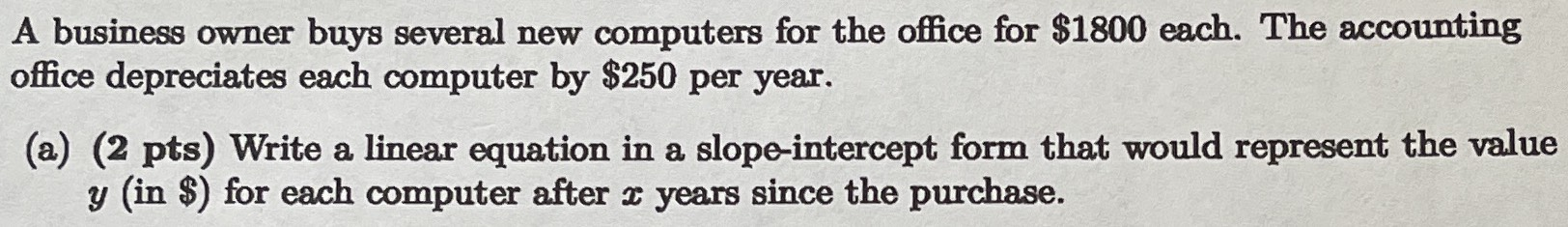### ¿Todavía tienes preguntas de matemáticas?

Pregunte a nuestros tutores expertos
Algebra
PreguntaA business owner buys several new computers for the office for $$\ 1800$$ each. The accounting office depreciates each computer by $$\ 250$$ per year.

(a) (2 pts) Write a linear equation in a slope-intercept form that would represent the value $$y ($$ in $$\ )$$ for each computer after $$x$$ years since the purchase.